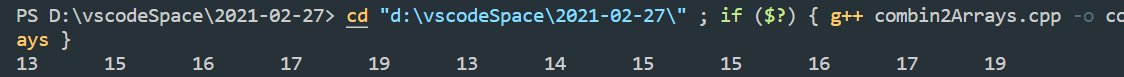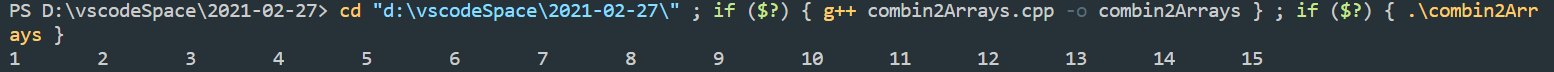• 主要为大家详细介绍了C++实现两个有序数组合并，文中示例代码介绍的非常详细，具有一定的参考价值，感兴趣的小伙伴们可以参考一下
• 合并两个有序数组 题目描述： 有两个排序的数组A1和A2，内存在A1的末尾有足够的空余空间容纳A2。请实现一个函数，把A2中的所有数字插入到A1中，并且所有的数字是排序的。 思路分析：从尾到头边比较，边移入正确的...

合并两个有序数组

题目描述：

有两个排序的数组A1和A2，内存在A1的末尾有足够的空余空间容纳A2。请实现一个函数，把A2中的所有数字插入到A1中，并且所有的数字是排序的。
思路分析：从尾到头边比较，边移入正确的位置，时间复杂度为O(n)

#include <iostream>
using namespace std;

void Combin2Array(int str1[], int length1, int str2[], int length2)
{
if (str1 == nullptr|| length1 <= 0 || str2 == nullptr || length2 <= 0)
return;
int len1 = 0;
for (int i = 0; i < length1; ++i) //使用for循环计算数组中实际的元素数目
{
if (str1[i])
len1++;
}
int newLength = len1+length2 - 1;//这是最新修改的地方！！！新数组的长度是两个数组非零元素数量之和
int len2 = length2 - 1;
len1--; //数组最后一个数字的序号为长度减一
while (len1 >= 0 && len2 >= 0)
{
if (str1[len1] < str2[len2])
{
str1[newLength--] = str2[len2--];
}
else
{
str1[newLength--] = str1[len1--];
}
}
//下面这部分是新增的，不可缺少,处理一个数组中的元素已经完全加入到新数组，另一个还没完的情况
while (len1 < 0 && len2 >= 0)
{
str1[newLength--] = str2[len2--];
}
while (len2 < 0 && len1 >= 0)
{
str1[newLength--] = str1[len1--];
}
}
int main()
{
int array1 = { 1, 3, 5, 7, 9 };
int array2[] = { 2, 4, 6, 8, 10 , 11, 12, 13, 14, 15};
//int array1 = {13, 15, 16, 17, 19 };
//int array2[] = { 2, 4, 6, 8, 10 , 11, 12, 13, 14, 15};
Combin2Array(array1, sizeof(array1) / sizeof(int), array2, sizeof(array2) / sizeof(int));

for (int i = 0; i < sizeof(array1) / sizeof(int); ++i)
{
if (array1[i])//增加判断条件，只输出非零元素！！！
{
cout << array1[i] << '\t';
}
}
/*基于范围的for循环
for (auto x :array1)
{
cout << x << '\t';
}
*/
return 0;
}

运行结果如下：展开全文c++ 数据结构
• 合并两个有序数组c++

千次阅读 2019-01-29 10:31:14
给定两个有序整数数组 nums1 和 nums2，将 nums2 合并到 nums1 中，使得 num1 成为一个有序数组。 说明: 初始化 nums1 和 nums2 的元素数量分别为 m 和 n。 你可以假设 nums1 有足够的空间（空间大小大于...

题目描述：

给定两个有序整数数组 nums1 nums2，将 nums2 合并到 nums1 使得 num1 成为一个有序数组。

说明:

• 初始化 nums1nums2 的元素数量分别为 mn
• 你可以假设 nums1 有足够的空间（空间大小大于或等于 m + n）来保存 nums2 中的元素。

示例:

输入:
nums1 = [1,2,3,0,0,0], m = 3
nums2 = [2,5,6],       n = 3

输出: [1,2,2,3,5,6]

思路1：

创建一个新的向量数组，很常规的一个思路，就是运用双指针法，依次比较即可。

代码：

class Solution {
public:
void merge(vector<int>& nums1, int m, vector<int>& nums2, int n) {

vector <int> array(m+n);
int k=0;
int i=0;
int j=0;
while(i!=m&&j!=n){
if(nums1[i]>nums2[j]){
array[k]=nums2[j];
k++;
j++;
}
else{
array[k]=nums1[i];
k++;
i++;
}
}
while(i<m){
array[k]=nums1[i];
i++;
k++;
}
while(j<n){
array[k]=nums2[j];
j++;
k++;
}
nums1.assign(array.begin(),array.end());
}
};

思路2：

不创建额外的数组，在nums1上进行操作，因为nums1数组空间足够大，所以可以从nums1数组的后面开始进行合并，大体写法和思路一差不多。

代码：

class Solution {
public:
void merge(vector<int>& nums1, int m, vector<int>& nums2, int n) {
int i=m-1;
int j=n-1;
int k=m+n-1;
while(i>=0&&j>=0){
if(nums1[i]>nums2[j]){
nums1[k]=nums1[i];
k--;
i--;
}
else{
nums1[k]=nums2[j];
k--;
j--;
}
}
while(i>=0){
nums1[k]=nums1[i];
k--;
i--;
}
while(j >= 0){
nums1[k] = nums2[j];
k--;
j--;
}
}
};

展开全文c++
• * 问题：有两个有序数组合并成一个有序数组。 * 分析：在内存中申请一个大小为两有序数组大小之和的新数组作为合并后的数组，从后往前把两个数组合并到新数组中。 * * MergeTwoSortedArrays.cpp - by ...

分享一个大牛的人工智能教程。零基础！通俗易懂！风趣幽默！希望你也加入到人工智能的队伍中来！请点击http://www.captainbed.net

/*
* 问题：有两个有序数组，合并成一个有序数组。
* 分析：在内存中申请一个大小为两有序数组大小之和的新数组作为合并后的数组，从后往前把两个数组合并到新数组中。
*
* MergeTwoSortedArrays.cpp - by LiveEveryDay
*/

#include <iostream>

using namespace std;

#define MAX 1024

int *MergeArray(int *arr1, int *arr2, int len1, int len2) {
if (arr1 == nullptr) {
return arr2;
}
if (arr2 == nullptr) {
return arr1;
}
if ((len1 + len2) > MAX) {
return nullptr;
}

int *mergedArray = new int[len1 + len2];
int p = len1 + len2 - 1;
int p1 = len1 - 1;
int p2 = len2 - 1;
while (p1 >= 0 && p2 >= 0) {
if (arr1[p1] > arr2[p2]) {
mergedArray[p--] = arr1[p1--];
} else {
mergedArray[p--] = arr2[p2--];
}
}
while (p1 >= 0) {
mergedArray[p--] = arr1[p1--];
}
while (p2 >= 0) {
mergedArray[p--] = arr2[p2--];
}

return mergedArray;
}

int main() {
int arr1 = {1, 3, 7, 8, 9, 11};
int arr2 = {-3, -1, 6, 8, 16, 19};
int *mergedArray = MergeArray(arr1, arr2, 6, 6);
for (int i = 0; i <= 6 + 6 - 1; i++) {
cout << *(mergedArray + i) << " ";
}
cout << endl;

return 0;
}

// Output:
/*
-3 -1 1 3 6 7 8 8 9 11 16 19
*/

展开全文程序设计 C++
• 合并两个有序数组 给定两个有序整数数组 nums1 和 nums2，将 nums2 合并到 nums1 中，使得 num1 成为一个有序数组。 说明: 初始化 nums1 和 nums2 的元素数量分别为 m 和 n。 你可以假设 nums1 有足够的空间（空间...

合并两个有序数组

给定两个有序整数数组 nums1 和 nums2，将 nums2 合并到 nums1 中，使得 num1 成为一个有序数组。

说明:

初始化 nums1 和 nums2 的元素数量分别为 m 和 n。
你可以假设 nums1 有足够的空间（空间大小大于或等于 m + n）来保存 nums2 中的元素。
示例:

输入:

nums1 = [1,2,3,0,0,0], m = 3
nums2 = [2,5,6],       n = 3
输出: [1,2,2,3,5,6]

解法1：自己写排序

class Solution {
public:
void merge(vector<int>& nums1, int m, vector<int>& nums2, int n) {
int sum=m+n;
int pos=0;//指向nums2准备存入的nums1的元素下标
if(n<1) ;//若nums2没有元素则不用整合
else
{
for(int i=0;i<sum&&n;i++)
{
if(nums2[pos]<=nums1[i] || i>=m)
{
for(int k=nums1.size()-1;k>i;k--)//将元素后移一位
{
nums1[k]=nums1[k-1];
}
nums1[i]=nums2[pos];//插入元素
pos++;
n--;
m++;
}
}
}
}
};

解法2：用内置的排序函数

class Solution {
public:
static bool cmp(const int &a,const int &b)
{
return a<b;
}
void merge(vector<int>& nums1, int m, vector<int>& nums2, int n) {
int sum=m+n;
int k=0;
for(int i=m;i<sum;i++)
{
nums1[i]=nums2[k];//将nums2的元素存入nums1然后排序
k++;
}
sort(nums1.begin(),nums1.end(),cmp);
}
};

展开全文leetcode c++
• 给你两个有序整数数组 nums1 和 nums2，请你将 nums2 合并到 nums1 中，使 nums1 成为一个有序数组。 初始化 nums1 和 nums2 的元素数量分别为 m 和 n 。你可以假设 nums1 的空间大小等于 m + n，这样它就有足够的...
• 合并两个有序数组 题目描述 现将数组2中的元素填充到数组1中，全都放到数组1元素的后面，当flag=0时，说明新数组当中元素已经排好顺序不需改变，若flag=1，则需进行数组排序，代码如下: ...
• 合并两个有序数组 思路 存在一个数组为空，则直接返回另一个非空数组。 都不为空 两个数组都非空：记两个数组分别为v1,v2，合并后的数组为vc，设置两个指针i、j分别指向数组v1,v2，令i=j=0， 若v1[i] <=...
• 给你两个有序整数数组 nums1 和 nums2，请你将 nums2 合并到 nums1 中，使 nums1 成为一个有序数组。 说明： 初始化 nums1 和 nums2 的元素数量分别为 m 和 n 。 你可以假设 nums1 有足够的空间（空间大小大于或等于...
• c++u语言合并两个有序数组 题目： 解题思路： 先把nums2插入到nums1，再进行排序。 代码实现： class Solution { public: void merge(vector<int>& nums1, int m, vector<int>& nums2, int ...
• 合并两个有序数组为新的有序数组 描述： 合并两个升序排序的整型数组为一个新的升序整型数组并输出。 输入格式: 首先输入第一个数组的数据个数后输入第一个数组按升序排序的数据，然后再输入第二个数组的数据个数，...java
• 给定两个有序整数数组 nums1 和 nums2，将 nums2 合并到 nums1 中，使得 num1 成为一个有序数组。 说明: 初始化 nums1 和 nums2 的元素数量分别为 m 和 n。 你可以假设 nums1 有足够的空间（空间大小大于或等于 m + ...
• 给定两个有序整数数组nums1和nums2，将nums2合并到nums1中，使得num1成为一个有序数组。 说明: 初始化nums1和nums2的元素数量分别为m和n。 你可以假设nums1有足够的空间（空间大小大于或等于m + n）来保存nums2中...
• 合并两个有序数组 给你两个有序整数数组 nums1 和 nums2，请你将 nums2 合并到 nums1 中，使 nums1 成为一个有序数组。 说明： 初始化 nums1 和 nums2 的元素数量分别为 m 和 n 。 你可以假设 nums1 有足够的空间...
• 给定两个有序整数数组 nums1 和 nums2，将 nums2 合并到 nums1 中，使得 num1 成为一个有序数组。 说明: 初始化 nums1 和 nums2 的元素数量分别为 m 和 n。 你可以假设 nums1 有足够的空间（空间大小大于或等于 m +...合并有序数组 C++
• 给定两个有序整数数组 nums1 和 nums2，合并nums1和nums2为有序数组 输入: nums1 = [1,2,3], m = 3 nums2 = [2,5,6], n = 3 输出: [1,2,2,3,5,6] **解题思路:**先求出两个数组的大小,开辟一个新数组来保存合并...
• 题目 有两个排序的数组A1和A2，内存在A1的末尾有足够多的空余空间容纳A2。请实现一个函数，把A2中的所有...课后相关题目-合并两个有序数组 //题目：有两个排序的数组A1和A2，内存在A1的末尾有足够多的空余空间容纳A...C++
• 给定两个有序整数数组 nums1 和 nums2，将 nums2 合并到 nums1 中，使得 num1 成为一个有序数组。 说明: 初始化 nums1 和 nums2 的元素数量分别为 m 和 n。 你可以假设 nums1 有足够的空间（空间大小大于或等于 m + ...C++ 合并两个有序数组
•合并两个数组
• 给定两个有序整数数组 nums1 和 nums2，将 nums2 合并到 nums1 中，使得 num1 成为一个有序数组。 说明: 初始化 nums1 和 nums2 的元素数量分别为 m 和 n。 你可以假设 nums1 有足够的空间（空间大小大于或等于 m...
• 这一方法将两个数组看作队列，每次从两个数组头部取出比较小的数字放到结果中。 class Solution { public: void merge(vector<int>& nums1, int m, vector<int>& nums2, int n) { int p1 = 0,p2 = 0;//我们为两个...c++
• 给你两个按 非递减顺序 排列的整数数组 nums1 和 nums2，另有两个整数 m 和 n ，分别表示 nums1 和 nums2 中的元素数目。 请你 合并 nums2 到 nums1 中，使合并后的数组同样按 非递减顺序 排列。 注意：最终，合并后...leetcode
• 代码题--C++--合并两个有序数组 题目描述： 给你两个有序整数数组nums1 和 nums2，请你将 nums2 合并到nums1中，使 nums1 成为一个有序数组。 说明： 初始化nums1 和 nums2 的元素数量分别为m 和 n 。 你可以...
• 时间复杂度O（2n）。 分别是比较：n，一一赋值：n class Solution { //19.51- public: void merge(vector<int>& nums1, int m, vector<int>& nums2, int n) { vector<... ...leetcode 算法
• 运行的有些慢，大佬们勿喷，毕竟是我这菜鸡自己想出来的思路，这方法是摁住其中一链表修改，遍历第一数字小的那个链表，只有当被修改的链表当前下一数字比list2_node的头数字大的时候才停止遍历，这...
• leetcode-88、合并两个有序数组 难度：简单 给你两个有序整数数组 nums1 和 nums2，请你将 nums2 合并到 nums1 中，使 nums1 成为一个有序数组。 说明： 初始化 nums1 和 nums2 的元素数量分别为 m 和 n 。 你可以...leetcode 算法
• 给出两个有序的整数数组A和B ，请将数组B 合并数组A 中，A变成一个有序的数组 注意： 可以假设A 数组有足够的空间存放B 数组的元素，A 和B 中初始的元素数目分别为m 和 n 解法 解法一：最笨的解法。创建一个新数组...数据结构
• 思路：因为两个数组为有序数组，所以我们可以从两个数组的末尾开始比较，取出两个数中的较大值，放入nums1的末尾。第一个while循环完成时，i 或者 j可能有一个最后大于0；当i&gt;0时，不需要管，因为最后结果...
• 题目传送：合并两个有序数组 解决办法： 三个指针（有点归并排序思想） void merge(vector<int>& nums1, int m, vector<int>& nums2, int n) { int end1 = m - 1; int end2 = n - 1; int......

合并两个有序数组c++c++ 订阅